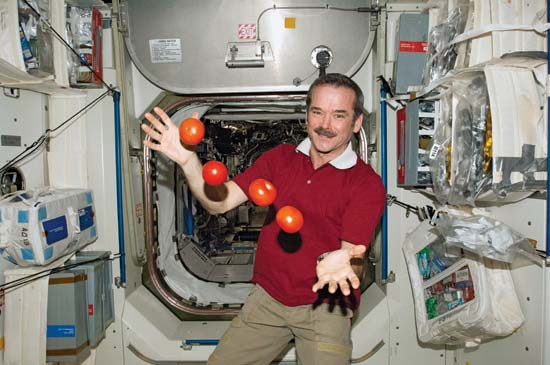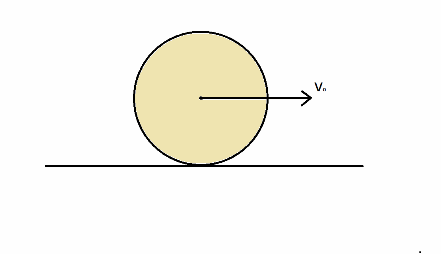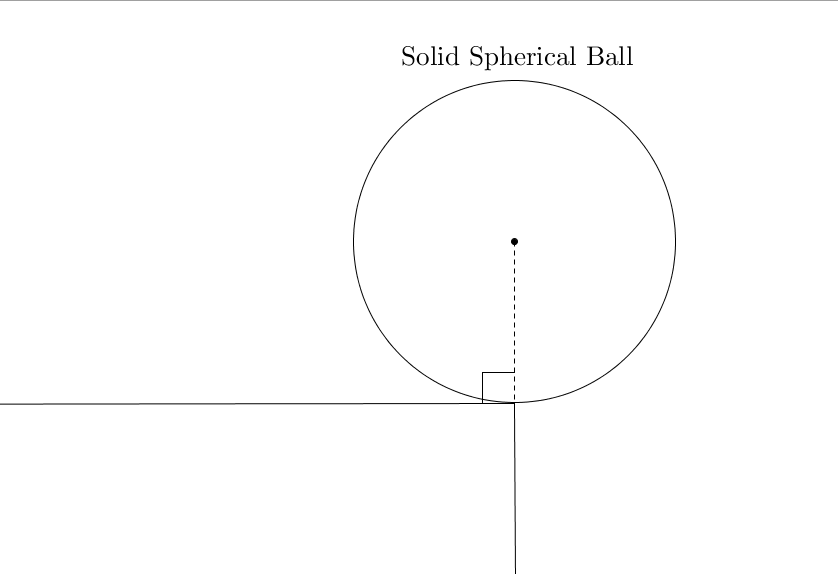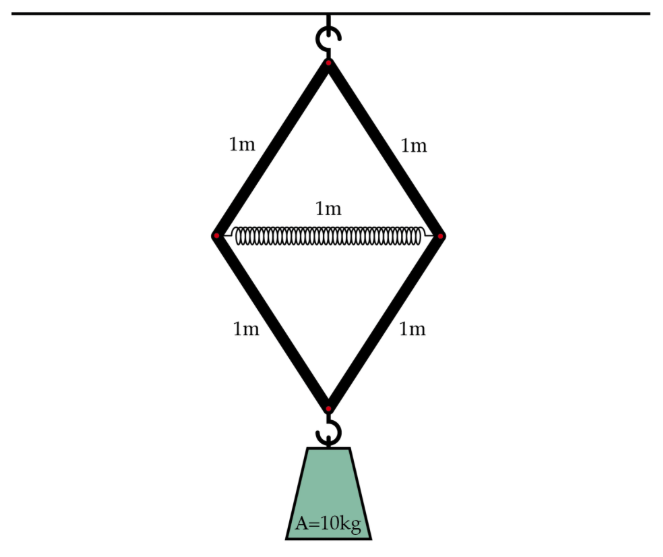Classical Mechanics

# 2D Dynamics: Level 3-4 ChallengesWhat is the period of rotation (in minutes) of the Earth so as to make any object weight-less on its equator?

Details and Assumptions

• The radius of the Earth is $R = 6 \times 10^{6}\text{ m}.$
• The gravitational acceleration is $g = 9.8\text{ m/s}^2.$A spherical ball of mass M and radius R is thrown along a rough horizontal surface so that initially, it slides with a linear speed $\displaystyle{\upsilon_0}$,but does not rotate. As it slides, it begins to spin and eventually rolls without slipping. The time taken to start rolling can be expressed as $\dfrac{a}{b} \times \dfrac{\upsilon_0}{\mu_k g}$ Where $\displaystyle{\mu_k}$ is the coefficient of kinetic friction between the surface and the ball .What is $\displaystyle{a+b}$?

Liked it? try some more

The Hyperloop is a hypothetical new fast transport system between cities, which works by launching pods that carry people through a very low air pressure tunnel. While riding in the Hyperloop, some coins fall out of your pocket, taking 1/3 of a second to hit the ground. How much more quickly would the coins have hit the ground if you were sitting still in seconds?

Details and assumptions

• The radius of the earth is $6370~\mbox{km}$.
• The Hyperloop travels at $300~\mbox{m/s}$.
• When sitting still relative to the Earth's surface, acceleration toward the Earth is $-9.8~\mbox{m/s}^2$.A solid spherical ball is placed carefully on the edge of a table in the position shown in the figure. The coefficient of static friction between the ball and the edge of the table is $0.5$. It is then given a very slight push. It begins to fall off the table.

Find the angle (in degrees) turned by the ball before it slips.

Details and Assumptions

• Find the angle with the vertical.A spring and four bars are hung from the ceiling in the configuration above. Each of the bars can pivot and rotate where it meets the other bar. The spring has a natural length of 1 m and a spring constant of 1000 N/m, while each of the bars has a length of 1 m and is effectively massless. A 10 kg weight is then hooked on to the bottom of this contraption at point A, causing the spring to squeeze. When the system comes to equilibrium, what is the length of the spring in meters?

Details and assumptions

• The acceleration of gravity is $-9.8~m/s^2$.
• The spring doesn't squeeze all that much, so you may assume that the vertical angles on the top and bottom remain at $60+\epsilon$ degrees, where $\epsilon$ is a small angle.
×